Next: Common problems Up: Using Maple Efficiently Previous: Functions versus Expressions

## Building Complicated Maple Commands

In the two previous sections, we've seen examples of how and when to use labels in Maple and how to choose between functions and expressions. In this section we consider the following situation. You have a problem whose solution requires several steps, but you aren't really interested in seeing the intermediate results. An efficient and elegant solution of problems like this can often be obtained by nesting Maple commands.

For example, in an earlier example we solved for the x values where the second derivative of a function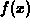vanished with the following command. Since a Maple command diff appears as an argument to the solve command, we describe such a command as a nested or compound Maple command.

```  > solve(diff(f(x),x)=0,x);
```

An alternative would be to break the calculation up into the following steps.

```  > f2 := diff(f(x),x,x);
```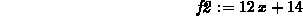```  > solve(f2=0,x);
```However, this solution requires more typing, including defining a label for the second derivative. If you don't need the second derivative for anything else, then it is wasteful to give it a label and the solution with a nested command is preferred.

The easiest way to build up a nested command is to start from the inside and work out. In the example, you would start with the diff command.

```  > diff(f(x),x,x);
```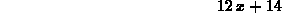Next, decide if you want to label this result for later use. If you only need it as an intermediate result in a longer calculation and not on its own, start building up a nested command. In the example, we don't need the second derivative ofexcept to find where it vanishes so the next step is to add the solve command, using the diff command as an argument. This leads to the following command and desired result.

```  > solve(diff(f(x),x,x)=0,x);
```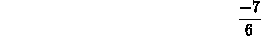This process of nesting commands can lead to Maple commands that look very complicated, for example the command

```  > subs(x=1/V,interp(map(s->1/s,V_vals),W_vals,x));
```

used in the section on labels. We now describe the steps in building up this command.

Recall that we had some data for V and W that we wanted to fit with an expression of the form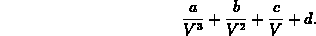The difficulty was that we wanted to use the Maple interp command, but it would only fit data to polynomial expressions. Note that the desired expression can be thought of as a polynomial in, so the first idea is to transform the V values to values of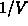. This can be done with the following commands. The commands defining the data as lists are repeated for convenience.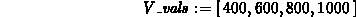```  > W_vals := [31.3,11.9,8.0,4.3];
``````  > map(s->1/s,V_vals);
```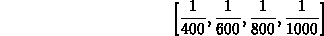The next step is to use the interp command to fit the modified data. Just modify the last command above, using the map command as the first argument to interp.

```  > interp(map(s->1/s,V_vals),W_vals,x);
```The result is really a polynomial in, so the last step is to substitutefor x. Again, we just add the subs command on the outside of the previous command.

```  > subs(x=1/V,interp(map(s->1/s,V_vals),W_vals,x));
```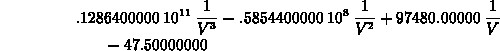As a final comment, there was no special reason we used x and s as ``dummy'' variables in the command. Any other variable names that we weren't using at the time would have worked.Next: Common problems Up: Using Maple Efficiently Previous: Functions versus Expressions

William W. Farr
Mon Aug 28 09:31:56 EDT 1995